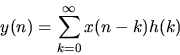## Z Transform Tutorial

Ele 541 Electronic Testing

Sequences

A discrete time signal consists of a sequence of numbers denoted by xn, x(n), or x(nT). Sequences of particular importance have been given special names.

• The unit pulse or impulse sequence is defined for all n by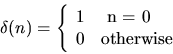• The unit step sequence is defined by• The exponential sequence is defined by•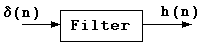Figure 1   A discrete time filter with a unit pulse input and and a response h(n).
Systems and Filters

The response of a system to a unit pulse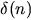is h(n).

Since the unit pulseis zero except when its argument, n, is zero, a sequence x(n) can be written as the weighted sum of a sequence of unit pulses.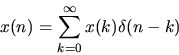where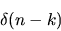is zero except when k=n. Since the response to a unit pulse delayed by k units is h(n-k), the response to the weighted sum of delayed unit pulses is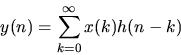This is the convolution summation. By changing variables you can show that the convolution summation can also be written as,